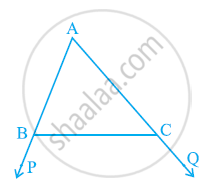# In the given figure sides AB and AC of ΔABC are extended to points P and Q respectively. Also, ∠PBC < ∠QCB. Show that AC > AB. - Mathematics

In the given figure sides AB and AC of ΔABC are extended to points P and Q respectively. Also, ∠PBC < ∠QCB. Show that AC > AB.#### Solution

In the given figure,

∠ABC + ∠PBC = 180° (Linear pair)

⇒ ∠ABC = 180° − ∠PBC ... (1)

Also,

∠ACB + ∠QCB = 180°

∠ACB = 180° − ∠QCB … (2)

As ∠PBC < ∠QCB,

⇒ 180º − ∠PBC > 180º − ∠QCB

⇒ ∠ABC > ∠ACB [From equations (1) and (2)]

⇒ AC > AB (Side opposite to the larger angle is larger.)

Concept: Inequalities in a Triangle
Is there an error in this question or solution?
Chapter 7: Triangles - Exercise 7.4 [Page 132]

#### APPEARS IN

NCERT Class 9 Maths
Chapter 7 Triangles
Exercise 7.4 | Q 2 | Page 132

Share